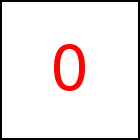# rotateMiddle Library Method

Short Description: Rotate around Objects Middle and given axis

Signature: t.rotateMiddle (ax,ay,az)
Group: Motion
Class: transition Class

Makes the object rotate around its center with the axis (ax,ay,az). ax..az must be numbers which can be integer or floating point. The vector (ax,ay,az) must be a unit vector, i.e. it must have length 1.

For example (ax,ay,az)=(0,0,1) makes the object rotate around the z-axis, i.e. inside the screen plane.

Per default a full rotation of 360 dregrees is performed. This can be changed using the rotatePart function. In order to rotate an object around an arbitrary point refer to rotate.

### Examples

 var a=taccgl.actor("testimg"); a.rotateMiddle(0,0,1).start(); RUN var a=taccgl.actor("testimg"); a.rotateMiddle(1,0,0).start(); RUN var a=taccgl.actor("pgMiddleColumnTable"); a.rotateMiddle(0,1,0).start(); RUN var a=taccgl.actor("pgMiddleColumnTable"); a.rotateMiddle(1/Math.sqrt(2),1/Math.sqrt(2),0).dur(5).start(); RUN var a=taccgl.actor("pgMiddleColumnTable"); a.rotateMiddle(1,1,0).dur(5).start(); RUN

WebGL™ is a trademark of the Khronos Group Inc.

Next Page:transition.rotatePart - Set range of rotation angle
Previous Page: transition.rotate - Rotate around given point and axis

# Motion

taccgl™ - HTML 5 Canvas Library